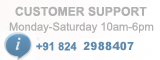# PIC Development Board

/*
* Project name:GSM INTERFACE
PIC Development Board
(c) Researchdesignlab.com
* Test configuration:
MCU: PIC16F877A
Dev.Board: PIC
Oscillator: 20.0 MHz
Software: MPLAB IDE v8.92(HI-TECH_C)
*/

#define _XTAL_FREQ 20000000 //crystal frequency of 20MHZ

char UART_Init(const long int baudrate)
{
unsigned int x;
x = (_XTAL_FREQ - baudrate*64)/(baudrate*64);
if(x>255)
{
x = (_XTAL_FREQ - baudrate*16)/(baudrate*16);
BRGH = 1; //High Baud Rate Select bit set to high
}
if(x<256)
{
SPBRG = x; //Writing SPBRG register
SYNC = 0; //Selecting Asynchronous Mode
SPEN = 1; //enables serial port
TRISC7 = 1;
TRISC6 = 1;
CREN = 1; //enables continuous reception
TXEN = 1; //enables continuous transmission
return 1;
}
return 0;
}

char UART_TX_Empty()
{
return TRMT; //Returns Transmit Shift Status bit
}

{
return RCIF; //Flag bit
}

{
while(!RCIF); //Waits for Reception to complete
return RCREG; //Returns the 8 bit data
}
void UART_Read_Text(char *Output, unsigned int length)//this function is used to read a text
{
int i;
for(int i=0;i<length;i++)
}

void UART_Write(char data) //this function is used to write a byte
{
while(!TRMT);
TXREG = data; //transmit register
}

void UART_Write_Text(char *text) //this function is used to write a string
{
int i;
for(i=0;text[i]!='\0';i++)
UART_Write(text[i]);
}
void main()
{
UART_Init(9600); //initialize the UART function
__delay_ms(1000); //provide the delay of 1s

while(1) //infinite loop
{
__delay_ms(1000); //provide a delay of 1s
UART_Write_Text("AT"); //attention command
UART_Write(13); //enter
UART_Write(10); //carriage return
__delay_ms(1000); //provide delay of 1s
UART_Write_Text("AT+CMGF=1"); //initialize the modem

UART_Write(13); //enter
UART_Write(10); //carriage return
__delay_ms(1000); //provide delay of 1s
UART_Write_Text("AT+CMGS=\"1234567890\""); //send a message

UART_Write(13); //enter
UART_Write(10); //carriage return
__delay_ms(1000); //provide delay of 1s
UART_Write_Text("GSM");//display on hyper terminal
UART_Write(13); //enter
UART_Write(10); //carriage return
__delay_ms(1000); //provide delay of 1s
UART_Write_Text("AT+CMGD=1");//delete the 1st message
UART_Write(13); //enter
UART_Write(10); //carriage return
__delay_ms(1000); //provide delay of 1s